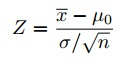# One Sample Z Test: How to Run One

Share on

Hypothesis Testing > One Sample Z Test

Watch the video for an example of a one sample z test, or read on below:
Watch the video for an example:

## How to Run a One Sample Z Test

A one sample z test is one of the most basic types of hypothesis test. In order to run a one sample z test, you work through several steps:

Step 1: State the Null Hypothesis. This is one of the common stumbling blocks--in order to make sense of your sample and have the one sample z test give you the right information you must make sure you’ve written the null hypothesis and alternate hypothesis correctly. For example, you might be asked to test the hypothesis that the mean weight gain of pregnant women was more than 30 pounds. Your null hypothesis would be: H0: μ = 30 and your alternate hypothesis would be H,sub>1: μ > 30.

Step 2: Use the z-formula to find a z-score.All you do is put in the values you are given into the formula. Your question should give you the sample mean (x̄), the standard deviation (σ), and the number of items in the sample (n). Your hypothesized mean (in other words, the mean you are testing the hypothesis for, or your null hypothesis) is μ0.

Example:
1,500 women followed the Atkin’s diet for a month. A random sample of 29 women gained an average of 6.7 pounds. Test the hypothesis that the average weight gain per woman for the month was over 5 pounds. The standard deviation for all women in the group was 7.1.
Z = 6.7 – 5 / (7.1/√29) = 1.289.

Step 3: Look up your z score in the z-table. The sample score above gives you an area of 0.8997. This area is your probability up to that point (i.e. the area to the left of your z-score). For this one sample z test, you want the area in the right tail, so subtract from 1:
1 – 0.8997 = 0.1003.

If you have difficulty figuring out if you have a left- or-right tailed test, see:
Left Tailed Test or Right Tailed Test? How to Decide in Easy Steps.

Next: several specific examples of one sample z tests (with answers):
Hypothesis Testing Examples

CITE THIS AS:
Stephanie Glen. "One Sample Z Test: How to Run One" From StatisticsHowTo.com: Elementary Statistics for the rest of us! https://www.statisticshowto.com/one-sample-z-test/
---------------------------------------------------------------------------Need help with a homework or test question? With Chegg Study, you can get step-by-step solutions to your questions from an expert in the field. Your first 30 minutes with a Chegg tutor is free!